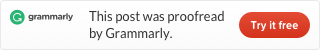Categories

# Leetcode 1. Two Sum (Java)

Source Url: https://leetcode.com/problems/two-sum/

Easy

Given an array of integers, return indices of the two numbers such that they add up to a specific target.

You may assume that each input would have exactly one solution, and you may not use the same element twice.

Example:

Given nums = [2, 7, 11, 15], target = 9,
Because nums + nums = 2 + 7 = 9, return [0, 1].

## Brute force approach

This is an easy problem, use the brute force approach is very easy. We can use double nesting loops, every layer from 0 to n-1. In the loop body, we test the two number’s sum with the target, if they equal return the value and quit (Because each input would have exactly one solution). And Because “may not use the same element twice”, you need to add an if statement to ensure it.

And this is the code.

Running time is 79ms, faster than only 6.2% java solutions.

Time complexity : O(n2), Space complexity : O(n)

## Hashtable two passes

Brute force approach uses double nesting loops, so it is so slow. We can store the numbers into a hashtable and use single layer loop to solve this problem.

In the first loop, we save the numbers into a hashtable, the key is the number itself, the value is the index of it.

Then in the second loop, we use the target to minus the current number, use this value to search in the hashtable. If the value exists in the hashtable, then we get our result, return it.

This is the code:

Running time is 4ms, beat 83.37% java solutions。

Time complexity : O(n)+O(n), Space complexity : O(n)

## Sort and approach from two ends

And there are other ways to solve this problem. This approach is that: First, we sort the array. Then we approach from two ends of the array, we get a left pointer and right pointer, start separately from left end and right end. Every iteration we check the numbers from left and right pointers, test their sum with the target. If the sum equals the target then return. If the sum larger than the target, we move the right pointer to left one step, else we move the left pointer to right one step. So we can use one loop to get the result, and we need a sort. Because we need the index, and the sort will ruin the origin order, so we need the additional array to store origin indexes.

This is the code:

Running time is 49ms,  time complexity is O(n log(n))+O(n), Space complexity is O(n).

## Hash-table and one pass

The essential of this approach is: We need to run the loop and at the same time store number to the hashtable, check number in the hashtable. If we get the right result return it and quit. If we don’t, put the current number into the hashtable.

This is the code:

Running time is 3ms faster than 99.75% java solutions. Time complexity is O(n), Space complexity is O(n).